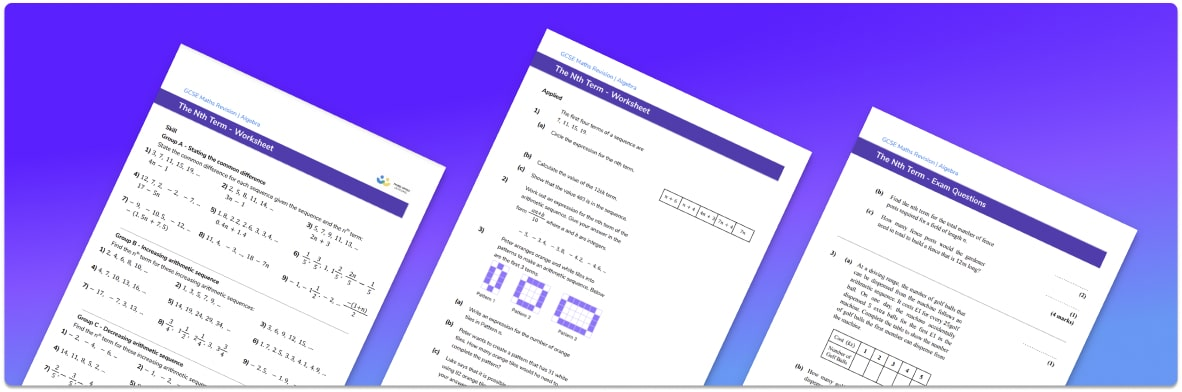# Nth Term Worksheet• Section 1 of the nth term worksheet contains 27 skills-based nth term questions, in 3 groups to support differentiation
• Section 2 contains 3 applied nth term questions with a mix of worded problems and deeper problem solving questions
• Section 3 contains 5 foundation and higher level GCSE exam practice questions
• Answers and a mark scheme for all questions are provided
• Questions follow variation theory with plenty of opportunities for students to work independently at their own level
• All questions created by fully qualified expert secondary maths teachers
• Suitable for GCSE maths revision for AQA, OCR and Edexcel exam boards

• This field is for validation purposes and should be left unchanged.

You can unsubscribe at any time (each email we send will contain an easy way to unsubscribe). To find out more about how we use your data, see our privacy policy.

### Nth Term at a glance

The nth term is a general rule for a number sequence. It is sometimes called the position-to-term rule.

The nth term of the sequence can be found for linear sequences by working out the common difference by subtracting a given term from the next term in the sequence, e.g. the 5th term subtracting the 4th term will give the common difference.

This common difference then gives the coefficient of n in the nth term formula. The remainder of the nth term can be found by considering how the sequence produced by the common difference multiplied by the term number n differs from the original sequence. For example, if the common difference was 4, we could use the term 4n to create a sequence which could then be compared with the original sequence and then adjusted accordingly.

The common difference can also be used to find missing terms of a sequence. For example, we could find the 1st term in the sequence by substituting n=1 into the sequence, the 10th term by substituting n=10, the 20th term by substituting n=20, the 50th term by substituting n=50 and the 100th term by substituting n=100 etc.

The nth term of a linear sequence can also be used to check if a number is in the sequence by setting the number equal to the nth term and solving the equation. If the solution is an integer, then the number is in the sequence. If the solution number is a decimal, then the number is not in the sequence.

We can find the nth term for different types of sequences such as quadratic sequences which involve square numbers.

Looking forward, students can progress with other sequences worksheets and on to additional algebra worksheets, for example a simplifying expressions worksheet or simultaneous equations worksheet.For more teaching and learning support on Algebra our GCSE maths lessons provide step by step support for all GCSE maths concepts.

## Do you have KS4 students who need more focused attention to succeed at GCSE?There will be students in your class who require individual attention to help them succeed in their maths GCSEs. In a class of 30, it’s not always easy to provide.

Help your students feel confident with exam-style questions and the strategies they’ll need to answer them correctly with our dedicated GCSE maths revision programme.

Lessons are selected to provide support where each student needs it most, and specially-trained GCSE maths tutors adapt the pitch and pace of each lesson. This ensures a personalised revision programme that raises grades and boosts confidence.

Find out more